# Magnetic field

Magnetic field

A magnetic field is a mathematical description of the magnetic influence of electric currents and magnetic materials. The magnetic field at any given point is specified by both a direction and a magnitude (or strength); as such it is a vector field.[nb 1] The magnetic field is most commonly defined in terms of the Lorentz force it exerts on moving electric charges. There are two separate but closely related fields to which the name 'magnetic field' can refer: a magnetic B field and a magnetic H field.

Magnetic fields are produced by moving electric charges and the intrinsic magnetic moments of elementary particles associated with a fundamental quantum property, their spin. In special relativity, electric and magnetic fields are two interrelated aspects of a single object, called the electromagnetic field tensor; the aspect of the electromagnetic field that is seen as a magnetic field is dependent on the reference frame of the observer. In quantum physics, the electromagnetic field is quantized and electromagnetic interactions result from the exchange of photons.

Magnetic fields have had many uses in ancient and modern society. The Earth produces its own magnetic field, which is important in navigation. Rotating magnetic fields are utilized in both electric motors and generators. Magnetic forces give information about the charge carriers in a material through the Hall effect. The interaction of magnetic fields in electric devices such as transformers is studied in the discipline of magnetic circuits.

## History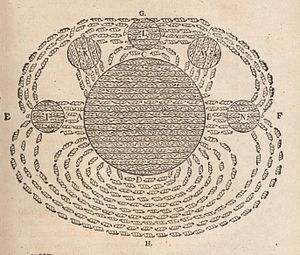One of the first drawings of a magnetic field, by René Descartes, 1644. It illustrated his theory that magnetism was caused by the circulation of tiny helical particles, "threaded parts", through threaded pores in magnets.

Although magnets and magnetism were known much earlier, one of the first descriptions of the magnetic field was produced in 1269 by the French scholar Petrus Peregrinus[nb 2] who mapped out the magnetic field on the surface of a spherical magnet using iron needles. Noting that the resulting field lines crossed at two points he named those points 'poles' in analogy to Earth's poles. Almost three centuries later, William Gilbert of Colchester replicated Petrus Peregrinus' work and was the first to state explicitly that Earth itself was a magnet. Gilbert's great work De Magnete was published in 1600 and helped to establish the study of magnetism as a science.

One of the first successful models of the magnetic field was developed in 1824 by Siméon-Denis Poisson (1781–1840). Poisson assumed that magnetism was due to 'magnetic charges' such that like 'magnetic charges' repulse while opposites attract. The model he created is exactly analogous to modern electrostatics with a magnetic H-field being produced by 'magnetic charges' in the same way that an electric field E-field is produced by electric charges. It predicts the correct H-field for permanent magnets. It predicts the forces between magnets. And, it predicts the correct energy stored in the magnetic fields.[nb 3]

Three remarkable discoveries though, would challenge Poisson's model. First, in 1819, Hans Christian Oersted discovered that an electric current generates a magnetic field encircling it. Then, André-Marie Ampère showed that parallel wires having currents in the same direction attract one another. Finally Jean-Baptiste Biot and Félix Savart discovered the Biot–Savart law which correctly predicts the magnetic field around any current-carrying wire.

Together, these discoveries suggested a model in which the magnetic B field of a material is produced by microscopic current loops. In this model, these current loops (called magnetic dipoles) would replace the dipoles of charge of the Poisson's model.[nb 4] No magnetic charges are needed which has the additional benefit of explaining why magnetic charge can not be isolated; cutting a magnet in half does not result in two separate poles but in two separate magnets, each of which has both poles.

The next decade saw two developments that help lay the foundation for the full theory of electromagnetism. In 1825, Ampère published his Ampère's law which like the Biot–Savart law correctly described the magnetic field generated by a steady current but was more general. And, in 1831, Michael Faraday showed that a changing magnetic field generates an encircling electric field and thereby demonstrated that electricity and magnetism are even more tightly knitted.

Between 1861 and 1865, James Clerk Maxwell developed and published a set of Maxwell's equations which explained and united all of classical electricity and magnetism. The first set of these equations was published in a paper entitled On Physical Lines of Force in 1861. The mechanism that Maxwell proposed to underlie these equations in this paper was fundamentally incorrect, which is not surprising since it predated the modern understanding even of the atom. Yet, the equations were valid although incomplete. He completed the set of Maxwell's equations in his later 1865 paper A Dynamical Theory of the Electromagnetic Field and demonstrated the fact that light is an electromagnetic wave. Thus, he theoretically unified not only electricity and magnetism but light as well. This fact was then later confirmed experimentally by Heinrich Hertz in 1887.

Even though the classical theory of electrodynamics was essentially complete with Maxwell's equations, the twentieth century saw a number of improvements and extensions to the theory. Albert Einstein, in his great paper of 1905 that established relativity, showed that both the electric and magnetic fields are part of the same phenomena viewed from different reference frames. (See moving magnet and conductor problem for details about the thought experiment that eventually helped Albert Einstein to develop special relativity.) Finally, the emergent field of quantum mechanics was merged with electrodynamics to form quantum electrodynamics or QED.

## Definitions, units, and measurement

Alternative names for the field B 
• Magnetic flux density
• Magnetic induction
• Magnetic field
Alternative names for the field H 
• Magnetic field intensity
• Magnetic field strength
• Magnetic field
• Magnetizing field

The term magnetic field is historically used to describe a magnetic H-field whereas other terms were used to describe a related magnetic B-field. Informally, and formally for some recent textbooks mostly in physics, the term 'magnetic field' is used to describe the magnetic B-field as well as or in place of H.[nb 5] There are many alternative names for both (see sidebar to right). To avoid confusion, this article uses B-field and H-field for these fields, and uses magnetic field where either or both fields apply.

The magnetic field can be defined in many equivalent ways based on the effects it has on its environment. For instance, a particle having an electric charge, q, and moving in a B-field with a velocity, v, experiences a force, F, called the Lorentz force. See Force on a charged particle below. Alternatively, the magnetic field can be defined in terms of the torque it produces on a magnetic dipole. See Torque on a magnet due to a B-field below.

The H-field is defined as a modification of B due to magnetic fields produced by material media. See H and B inside and outside of magnetic materials below for the relationship between B and H. Outside of a material (i.e., in vacuum) the B and H fields are indistinguishable. (They only differ by a multiplicative constant.) Inside a material, though, they may differ in relative magnitude and even direction. Often, though, they differ only by a material dependent multiplicative constant.

The B-field is measured in teslas in SI units and in gauss in cgs units. (1 tesla = 10,000 gauss). The SI unit of tesla is equivalent to (newton·second)/(coulomb·metre).[nb 6] The H-field is measured in ampere-turn per metre (A/m) in SI units, and in oersteds (Oe) in cgs units.

Devices used to measure the local magnetic field are called magnetometers. Important classes of magnetometers include using a rotating coil, Hall effect magnetometers, NMR magnetometers, SQUID magnetometers, and fluxgate magnetometers. The magnetic fields of distant astronomical objects are measured through their effects on local charged particles. For instance, electrons spiraling around a field line produce synchrotron radiation which is detectable in radio waves.

The smallest precision level for a magnetic field measurement is on the order of attoteslas (10−18 tesla); the largest magnetic field produced in a laboratory is 2,800 T (VNIIEF in Sarov, Russia, 1998). The magnetic field of some astronomical objects such as magnetars are much higher; magnetars range from 0.1 to 100 GT (108 to 1011 T). See orders of magnitude (magnetic field).

## Magnetic field lines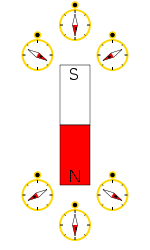Compasses reveal the direction of the local magnetic field. As seen here, the magnetic field points towards a magnet's south pole and away from its north pole.

Mapping the magnetic field of an object is simple in principle. First, measure the strength and direction of the magnetic field at a large number of locations. Then, mark each location with an arrow (called a vector) pointing in the direction of the local magnetic field with a length proportional to the strength of the magnetic field.

A simpler way to visualize the magnetic field is to 'connect' the arrows to form "magnetic field lines". Magnetic field lines make it much easier to visualize and understand the complex mathematical relationships underlying magnetic field. If done carefully, a field line diagram contains the same information as the vector field it represents. The magnetic field can be estimated at any point on a magnetic field line diagram (whether on a field line or not) using the direction and density of nearby magnetic field lines.[nb 7] A higher density of nearby field lines indicates a stronger magnetic field.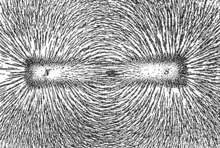The direction of magnetic field lines represented by the alignment of iron filings sprinkled on paper placed above a bar magnet. The mutual attraction of opposite poles of the iron filings results in the formation of elongated clusters of filings along "field lines". The field is not precisely the same as around the isolated magnet; the magnetization of the filings alters the field somewhat.[citation needed]

Various phenomena have the effect of "displaying" magnetic field lines as though the field lines are physical phenomena. For example, iron filings placed in a magnetic field line up to form lines that correspond to 'field lines'. Magnetic fields "lines" are also visually displayed in polar auroras, in which plasma particle dipole interactions create visible streaks of light that line up with the local direction of Earth's magnetic field. However, field lines are a visual and conceptual aid only and are no more real than (for example) the contour lines (constant altitude) on a topographic map. They do not exist in the actual field; a different choice of mapping scale could show twice as many "lines" or half as many.

Field lines can be used as a qualitative tool to visualize magnetic forces. In ferromagnetic substances like iron and in plasmas, magnetic forces can be understood by imagining that the field lines exert a tension, (like a rubber band) along their length, and a pressure perpendicular to their length on neighboring field lines. 'Unlike' poles of magnets attract because they are linked by many field lines; 'like' poles repel because their field lines do not meet, but run parallel, pushing on each other.

Most physical phenomena that "display" magnetic field lines do not include which direction along the lines that the magnetic field is in. A compass, though, reveals that magnetic field lines outside of a magnet point from the north pole (compass points away from north pole) to the south (compass points toward the south pole). The magnetic field of a straight current-carrying wire encircles the wire with a direction that depends on the direction of the current and that can be measured with a compass as well.

### B-field lines never end

Field lines are a useful way to represent any vector field and often reveal sophisticated properties of fields quite simply. One important property of the B-field revealed this way is that magnetic B field lines neither start nor end (mathematically, B is a solenoidal vector field); a field line either extends to infinity or wraps around to form a closed curve.[nb 8] To date no exception to this rule has been found. (See magnetic monopole below.)

Magnetic field lines exit a magnet near its north pole and enter near its south pole, but inside the magnet B-field lines continue through the magnet from the south pole back to the north.[nb 9] If a B-field line enters a magnet somewhere it has to leave somewhere else; it is not allowed to have an end point. Magnetic poles, therefore, always come in N and S pairs. Cutting a magnet in half results in two separate magnets each with both a north and a south pole.

More formally, since all the magnetic field lines that enter any given region must also leave that region, subtracting the 'number'[nb 10] of field lines that enter the region from the number that exit gives identically zero. Mathematically this is equivalent to:$\oint_S \mathbf{B} \cdot \mathrm{d}\mathbf{A} = 0$,

where the integral is a surface integral over the closed surface S (a closed surface is one that completely surrounds a region with no holes to let any field lines escape). Since dA points outward, the dot product in the integral is positive for B-field pointing out and negative for B-field pointing in.

There is also a corresponding differential form of this equation covered in Maxwell's equations below.

### H-field lines begin and end near magnetic poles

Unlike B-field lines, which never end, the H-field lines due to a magnetic material begin in a region(s) of the magnet called the north pole pass through the magnet and/or outside of the magnet and ends in a different region of the material called the south pole. Near the north pole, therefore, all H-field lines point away from the north pole (whether inside the magnet or out) while near the south pole (whether inside the magnet or out) all H-field lines point toward the south pole. (The B-field lines, for comparison, form a closed loop going from south to north inside the magnet and from north to south outside the magnet)

The H-field, therefore, is analogous to the electric field E which starts at a positive charge and ends at a negative charge. It is tempting, therefore, to model magnets in terms of magnetic charges localized near the poles. Unfortunately, this model is incorrect; for instance, it often fails when determining the magnetic field inside of magnets. (See "Non-uniform magnetic field causes like poles to repel and opposites to attract" below.)

Outside a material, though, the H-field is identical to the B-field (to a multiplicative constant) so that in many cases the distinction can be ignored. This is particularly true for magnetic fields, such as those due to electric currents, that are not generated by magnetic materials.

### Magnetic monopole (hypothetical)

A magnetic monopole is a hypothetical particle (or class of particles) that has, as its name suggests, only one magnetic pole (either a north pole or a south pole). In other words, it would possess a "magnetic charge" analogous to an electric charge. Magnetic field lines would start or end on magnetic monopoles, so if they exist, they would give exceptions to the rule that magnetic field lines neither start nor end.

Modern interest in this concept stems from particle theories, notably Grand Unified Theories and superstring theories, that predict either the existence, or the possibility, of magnetic monopoles. These theories and others have inspired extensive efforts to search for monopoles. Despite these efforts, no magnetic monopole has been observed to date.[nb 11]

In recent research, materials known as spin ices can simulate monopoles, but do not contain actual monopoles.[citation needed]

## The magnetic field and permanent magnets

Permanent magnets are objects that produce their own persistent magnetic fields. They are made of ferromagnetic materials, such as iron and nickel, that have been magnetized, and they have both a north and a south pole.

### Magnetic field of permanent magnets

The magnetic field of permanent magnets can be quite complicated, especially near the magnet. The B field of a small[nb 12] straight magnet is proportional to the magnet's strength (called its magnetic dipole moment m). The equations are non-trivial and also depend on the distance from the magnet and the orientation of the magnet. For simple magnets, m points in the direction of a line drawn from the south to the north pole of the magnet. Flipping a bar magnet is equivalent to rotating its m by 180 degrees.

It is sometimes useful to model the force and torques between two magnets as due to magnetic poles repelling or attracting each other in the same manner as the Coulomb force between electric charges. In this model, a magnetic H-field is produced by magnetic charges that are 'smeared' around each pole. A north pole therefore feels a force in the direction of the H-field while the force on the south pole is opposite to the H-field.

Unfortunately, the concept of poles of 'magnetic charge' does not accurately reflect what happens inside a magnet (see ferromagnetism). Magnetic charges do not exist. Magnetic poles cannot exist apart from each other; all magnets have north/south pairs which cannot be separated without creating two magnets each having a north/south pair. Finally, magnetic charge fails to account for magnetism that is produced by electric currents nor the force that a magnetic field applies to moving electric charges.

The more physically correct description of magnetism involves atomic sized loops of current distributed throughout the magnet.

### Non-uniform magnetic field causes like poles to repel and opposites to attract

The force between two small magnets is quite complicated and depends on the strength and orientation of both magnets and the distance and direction of the magnets relative to each other. The force is particularly sensitive to rotations of the magnets due to magnetic torque. The force on each magnet depends on its magnetic moment and the magnetic field B[nb 13] of the other.

To understand the force between magnets and to generalize it to other cases, it is useful to examine the magnetic charge model given above (with the caveats given above as well). In this model, the H-field of the first magnet pushes and pulls on the magnetic charges near both poles of the second magnet. If the H-field due to the first magnet is the same at both poles of the second magnet then there is no net force on that magnet since the force is opposite for opposite poles. The magnetic field is not the same, though; the magnetic field is significantly stronger near the poles of a magnet. In this nonuniform magnetic field, each pole sees a different field and is subject to a different force. This difference in the two forces moves the magnet in the direction of increasing magnetic field and may also cause a net torque.

This is a specific example of a general rule that magnets are attracted (or repulsed depending on the orientation of the magnet) into regions of higher magnetic field. Any non-uniform magnetic field whether caused by permanent magnets or by electric currents will exert a force on a small magnet in this way.

Mathematically, the force on a small magnet having a magnetic moment m due to a magnetic field B is:$\mathbf{F} = \mathbf{\nabla} \left(\mathbf{m}\cdot\mathbf{B}\right),$

where the gradient is the change of the quantity m · B per unit distance and the direction is that of maximum increase of m · B. To understand this equation, note that the dot product m · B = mBcos(θ), where m and B represent the magnitude of the m and B vectors and θ is the angle between them. If m is in the same direction as B then the dot product is positive and the gradient points 'uphill' pulling the magnet into regions of higher B-field (more strictly larger m · B). This equation is strictly only valid for magnets of zero size, but is often a good approximation for not too large magnets. The magnetic force on larger magnets is determined by dividing them into smaller regions having their own m then summing up the forces on each of these regions.

### Torque on a magnet due to a B-field

Magnetic torque on a magnet due to an external magnetic field can be observed by placing two magnets near each other while allowing one to rotate. Magnetic torque is used to drive simple electric motors. In one simple motor design, a magnet is fixed to a freely rotating shaft and subjected to a magnetic field from an array of electromagnets. By continuously switching the electric current through each of the electromagnets, thereby flipping the polarity of their magnetic fields, like poles are kept next to the rotor; the resultant torque is transferred to the shaft. See Rotating magnetic fields below.

Magnetic torque τ tends to align a magnet's poles with the B-field lines (since m is in the direction of the poles this is equivalent to saying that it tends to align m in the same direction as B). This is why the magnetic needle of a compass points toward earth's north pole. By definition, the direction of the Earth's local magnetic field is the direction in which the north pole of a compass (or of any magnet) tends to point.

Mathematically, the torque τ on a small magnet is proportional both to the applied B-field and to the magnetic moment m of the magnet:$\boldsymbol{\tau}=\mathbf{m}\times\mathbf{B}, \,$

where × represents the vector cross product. Note that this equation includes all of the qualitative information included above. There is no torque on a magnet if m is in the same direction as B. (The cross product is zero for two vectors that are in the same direction.) Further, all other orientations feel a torque that twists them toward the direction of B.

## The magnetic field and electric currents

Currents of electric charges both generate a magnetic field and feel a force due to magnetic B-fields.

### Magnetic field due to moving charges and electric currents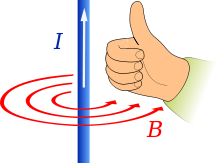Right hand grip rule: current (I) flowing through a conductor in the direction indicated by the white arrow produces a magnetic field (B) around the conductor as shown by the red arrows.

All moving charged particles produce magnetic fields. Moving point charges, such as electrons, produce complicated but well known magnetic fields that depend on the charge, velocity, and acceleration of the particles.

Magnetic field lines form in concentric circles around a cylindrical current-carrying conductor, such as a length of wire. The direction of such a magnetic field can be determined by using the "right hand grip rule" (see figure at right). The strength of the magnetic field decreases with distance from the wire. (For an infinite length wire the strength decreases inversely proportional to the distance.)

Bending a current-carrying wire into a loop concentrates the magnetic field inside the loop while weakening it outside. Bending a wire into multiple closely spaced loops to form a coil or "solenoid" enhances this effect. A device so formed around an iron core may act as an electromagnet, generating a strong, well-controlled magnetic field. An infinitely long cylindrical electromagnet has a uniform magnetic field inside, and no magnetic field outside. A finite length electromagnet produces a magnetic field that looks similar to that produced by a uniform permanent magnet, with its strength and polarity determined by the current flowing through the coil.

The magnetic field generated by a steady current I (a constant flow of electric charges in which charge is neither accumulating nor depleting at any point)[nb 14] is described by the Biot–Savart law:$\mathbf{B} = \frac{\mu_0I}{4\pi}\int\frac{d\boldsymbol{\ell} \times \mathbf{\hat r}}{r^2},$

where the integral sums over the wire length where vector d is the direction of the current, μ0 is the magnetic constant, r is the distance between the location of d and the location at which the magnetic field is being calculated, and is a unit vector in the direction of r.

A slightly more general[nb 15] way of relating the current I to the B-field is through Ampère's law:$\oint \mathbf{B} \cdot d\boldsymbol{\ell} = \mu_0 I_{\mathrm{enc}},$

where the line integral is over any arbitrary loop and Ienc is the current enclosed by that loop. Ampère's law is always valid for steady currents and can be used to calculate the B-field for certain highly symmetric situations such as an infinite wire or an infinite solenoid.

In a modified form that accounts for time varying electric fields, Ampère's law is one of four Maxwell's equations that describe electricity and magnetism.

### Force on moving charges and current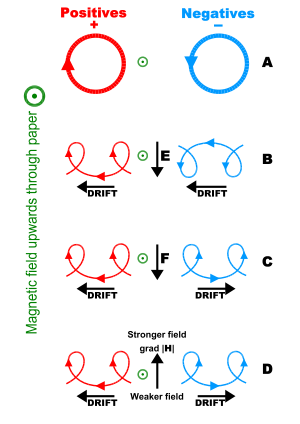Charged particle drifts in a magnetic field with (A) no net force, (B) an electric field, E, (C) a charge independent force, F (e.g. gravity), and (D) an inhomogeneous magnetic field, grad H.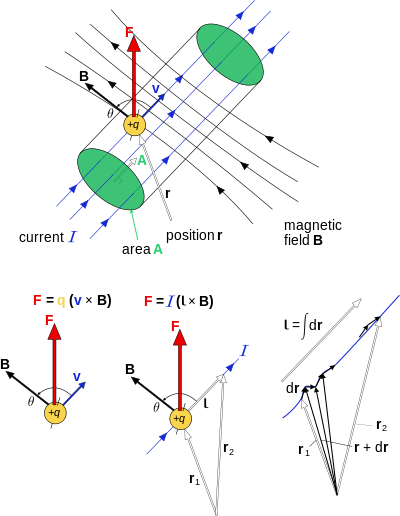Magnetic force on charged particles and currents, shown in 3d. The electric current shown here is conventional, the real current would be a charge of –q flowing in exactly the opposite direction. Only one charge carrier is shown to prevent cluttering the diagram. r1 is the position of entry into the field B, r2 is the exit. The vector l is the integral (sum) of all all infinitesimal vectors dr from r1 to r2.

#### Force on a charged particle

A charged particle moving in a B-field experiences a sideways force that is proportional to the strength of the magnetic field, the component of the velocity that is perpendicular to the magnetic field and the charge of the particle. This force is known as the Lorentz force, and is given by$\mathbf{F} = q \mathbf{v} \times \mathbf{B},$

where F is the force, q is the electric charge of the particle, v is the instantaneous velocity of the particle, and B is the magnetic field (in teslas).

The Lorentz force is always perpendicular to both the velocity of the particle and the magnetic field that created it. When a charged particle moves in a static magnetic field it will trace out a helical path in which the helix axis is parallel to the magnetic field and in which the speed of the particle will remain constant. Because the magnetic force is always perpendicular to the motion, the magnetic field can do no work on an isolated charge. It can only do work indirectly, via the electric field generated by a changing magnetic field. It is often claimed that the magnetic force can do work to a non-elementary magnetic dipole, or to charged particles whose motion is constrained by other forces, but this is incorrect because the work in those cases is performed by the electric forces of the charges deflected by the magnetic field.

#### Force on current-carrying wire

The force on a current carrying wire is similar to that of a moving charge as expected since a charge carrying wire is a collection of moving charges. A current carrying wire feels a sideways force in the presence of a magnetic field. The Lorentz force on a macroscopic current is often referred to as the Laplace force. Consider a conductor of length l and area of cross section A and has charge q which is due to electric current i .If a conductor is placed in a magnetic field of induction B which makes an angle θ (theta) with the velocity of charges in the conductor which has i current flowing in it. then force exerted due to small particle q is$\mathbf{F} = q \mathbf{v}\sin(\theta),$

then for n number of charges it has

N = nlA

then force exerted on the body is$f=\mathbf{F}N=q \mathbf{v}\sin(\theta)(nlA)$

but

nqvA = i

that is$f=\mathbf{B}il\sin(\theta)$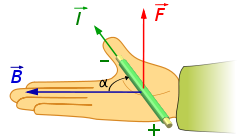The right-hand rule: Pointing the thumb of the right hand in the direction of the conventional current and the fingers in the direction of the B-field the force on the current points out of the palm. The force is reversed for a negative charge.

#### Direction of force

The direction of force on a charge or a current can be determined by a mnemonic known as the right-hand rule. See the figure on the left. Using the right hand and pointing the thumb in the direction of the moving positive charge or positive current and the fingers in the direction of the magnetic field the resulting force on the charge points outwards from the palm. The force on a negatively charged particle is in the opposite direction. If both the speed and the charge are reversed then the direction of the force remains the same. For that reason a magnetic field measurement (by itself) cannot distinguish whether there is a positive charge moving to the right or a negative charge moving to the left. (Both of these cases produce the same current.) On the other hand, a magnetic field combined with an electric field can distinguish between these, see Hall effect below.

An alternative mnemonic to the right hand rule is Fleming's left hand rule.

## H and B inside and outside of magnetic materials

The formulas derived for the magnetic field above are correct when dealing with the entire current. A magnetic material placed inside a magnetic field, though, generates its own bound current which can be a challenge to calculate. (This bound current is due to the sum of atomic sized current loops and the spin of the subatomic particles such as electrons that make up the material.) The H-field as defined above helps factor out this bound current; but in order to see how, it helps to introduce the concept of magnetization first.

### Magnetization

The magnetization field M represents how strongly a region of material is magnetized. For a uniform magnet, the magnetization is equal to its magnetic moment, m, divided by its volume. More generally, the magnetization of a region is defined as net magnetic dipole moment per unit volume of that region. Since the SI unit of magnetic moment is ampere-turn meter2, the SI unit of magnetization M is ampere-turn per meter which is identical to that of the H-field.

The magnetization M field of a region points in the direction of the average magnetic dipole moment in the region and is in the same direction as the local B-field it produces. Therefore, M field lines move from near the south pole of a magnet to near its north. Unlike B, magnetization only exists inside a magnetic material. Therefore, magnetization field lines begin and end near magnetic poles.

The physically correct way to represent magnetization is to add all of the currents of the dipole moments that produce the magnetization. See Magnetic dipoles below and magnetic poles vs. atomic currents for more information. The resultant current is called bound current and is the source of the magnetic field due to the magnet. Given the definition of the magnetic dipole, the magnetization field follows a similar law to that of Ampere's law:$\oint \mathbf{M} \cdot d\boldsymbol{\ell} = I_{\mathrm{b}},$

where the integral is a line integral over any closed loop and Ib is the 'bound current' enclosed by that closed loop.

It is also possible to model the magnetization in terms of magnetic charge in which magnetization begins at and ends at bound 'magnetic charges'. If a given region, therefore, has a net positive 'magnetic charge' then it will have more magnetic field lines entering it than leaving it. Mathematically this is equivalent to:$\oint_S \mu_0 \mathbf{M} \cdot \mathrm{d}\mathbf{A} = -q_M$,

where the integral is a closed surface integral over the closed surface S and qM is the 'magnetic charge' (in units of magnetic flux) enclosed by S. (A closed surface completely surrounds a region with no holes to let any field lines escape.) The negative sign occurs because, like B inside a magnet, the magnetization field moves from south to north.

### H-field and magnetic materials

The H-field is defined as:$\mathbf{H}\ \equiv \ \frac{\mathbf{B}}{\mu_0}-\mathbf{M},$(definition of H in SI units)

With this definition, Ampere's law becomes:$\oint \mathbf{H} \cdot d\boldsymbol{\ell} = \oint (\frac{\mathbf{B}}{\mu_0} - \mathbf{M}) \cdot d\boldsymbol{\ell} = I_{\mathrm{tot}}- I_{\mathrm{b}} = I_{\mathrm{f}},$

where If represents the 'free current' enclosed by the loop so that the line integral of H does not depend at all on the bound currents. For the differential equivalent of this equation see Maxwell's equations. Ampere's law leads to the boundary condition$H_{1,\parallel} - H_{2,\parallel} = \mathbf{K}_\text{f},$

where Kf is the surface free current density.

Similarly, a surface integral of H over any closed surface is independent of the free currents and picks out the 'magnetic charges' within that closed surface:$\oint_S \mu_0 \mathbf{H} \cdot \mathrm{d}\mathbf{A} = \oint_S (\mathbf{B}- \mu_0 \mathbf{M})\cdot \mathrm{d}\mathbf{A}= (0 - (-q_M)) = q_M,$

which does not depend on the free currents.

The H-field, therefore, can be separated into two[nb 16] independent parts:$\mathbf{H} = \mathbf{H}_0 + \mathbf{H}_d, \,$

where H0 is the applied magnetic field due only to the free currents and Hd is the demagnetizing field due only to the bound currents.

The magnetic H-field, therefore, re-factors the bound current in terms of 'magnetic charges'. The H field lines loop only around 'free current' and, unlike the magnetic B field, begins and ends at near magnetic poles as well.

### Magnetism

Most materials respond to an applied B-field by producing their own magnetization M and therefore their own B-field. Typically, the response is very weak and exists only when the magnetic field is applied. The term 'magnetism' describes how materials respond on the microscopic level to an applied magnetic field and is used to categorize the magnetic phase of a material. Materials are divided into groups based upon their magnetic behavior:

• Diamagnetic materials produce a magnetization that opposes the magnetic field.
• Paramagnetic materials produce a magnetization in the same direction as the applied magnetic field.
• Ferromagnetic materials and the closely related ferrimagnetic materials and antiferromagnetic materials can have a magnetization independent of an applied B-field with a complex relationship between the two fields.
• Superconductors (and ferromagnetic superconductors) are materials that are characterized by perfect conductivity below a critical temperature and magnetic field. They also are highly magnetic and can be perfect diamagnets below a lower critical magnetic field. Superconductors often have a broad range of temperatures and magnetic fields (the so named mixed state) for which they exhibit a complex hysteretic dependence of M on B.

In the case of paramagnetism and diamagnetism, the magnetization M is often proportional to the applied magnetic field such that:$\mathbf{B} = \mu \mathbf{H},$

where μ is a material dependent parameter called the permeability. In some cases the permeability may be a second rank tensor so that H may not point in the same direction as B. These relations between B and H are examples of constitutive equations. However, superconductors and ferromagnets have a more complex B to H relation, see magnetic hysteresis.

## Energy stored in magnetic fields

Energy is needed to generate a magnetic field both to work against the electric field that a changing magnetic field creates and to change the magnetization of any material within the magnetic field. For non-dispersive materials this same energy is released when the magnetic field is destroyed so that this energy can be modeled as being stored in the magnetic field.

For linear, non-dispersive, materials (such that B = μH where μ is frequency-independent), the energy density is:$u = \frac{\mathbf{B}\cdot\mathbf{B}}{2\mu} = \frac{\mu\mathbf{H}\cdot\mathbf{H}}{2}.$

If there are no magnetic materials around then μ can be replaced by μ0. The above equation cannot be used for nonlinear materials, though; a more general expression given below must be used.

In general, the incremental amount of work per unit volume δW needed to cause a small change of magnetic field δB is:$\delta W = \mathbf{H}\cdot\delta\mathbf{B}.$

Once the relationship between H and B is known this equation is used to determine the work needed to reach a given magnetic state. For hysteretic materials such as ferromagnets and superconductors the work needed will also depend on how the magnetic field is created. For linear non-dispersive materials, though, the general equation leads directly to the simpler energy density equation given above.

## Electromagnetism: the relationship between magnetic and electric fields

### Faraday's Law: Electric force due to a changing B-field

A changing magnetic field, such as a magnet moving through a conducting coil, generates an electric field (and therefore tends to drive a current in the coil). This is known as Faraday's law and forms the basis of many electrical generators and electric motors.$\mathcal{E} = - \frac{d\Phi_m}{dt},$

where$\scriptstyle\mathcal{E}$ is the electromotive force (or EMF, the voltage generated around a closed loop) and Φm is the magnetic flux—the product of the area times the magnetic field normal to that area. (This definition of magnetic flux is why B is often referred to as magnetic flux density.)

The negative sign is necessary and represents the fact that any current generated by a changing magnetic field in a coil produces a magnetic field that opposes the change in the magnetic field that induced it. This phenomenon is known as Lenz's Law.

This integral formulation of Faraday's law can be converted[nb 17] into a differential form, which applies under slightly different conditions. This form is covered as one of Maxwell's equations below.

### Maxwell's correction to Ampère's Law: The magnetic field due to a changing electric field

Similar to the way that a changing magnetic field generates an electric field, a changing electric field generates a magnetic field. This fact is known as 'Maxwell's correction to Ampère's law'. Maxwell's correction to Ampère's Law bootstrap together with Faraday's law of induction to form electromagnetic waves, such as light. Thus, a changing electric field generates a changing magnetic field which generates a changing electric field again.

Maxwell's correction to Ampère law is applied as an additive term to Ampere's law given above. This additive term is proportional to the time rate of change of the electric flux and is similar to Faraday's law above but with a different and positive constant out front. (The electric flux through an area is proportional to the area times the perpendicular part of the electric field.)

This full Ampère law including the correction term is known as the Maxwell–Ampère equation. It is not commonly given in integral form because the effect is so small that it can typically be ignored in most cases where the integral form is used. The Maxwell term is critically important in the creation and propagation of electromagnetic waves. These, though, are usually described using the differential form of this equation given below.

### Maxwell's equations

Like all vector fields the B-field has two important mathematical properties that relates it to its sources. (For magnetic fields the sources are currents and changing electric fields.) These two properties, along with the two corresponding properties of the electric field, make up Maxwell's Equations. Maxwell's Equations together with the Lorentz force law form a complete description of classical electrodynamics including both electricity and magnetism.

The first property is the divergence of a vector field A,  · A which represents how A 'flows' outward from a given point. As discussed above, a B-field line never starts or ends at a point but instead forms a complete loop. This is mathematically equivalent to saying that the divergence of B is zero. (Such vector fields are called solenoidal vector fields.) This property is called Gauss's law for magnetism and is equivalent to the statement that there are no magnetic charges or magnetic monopoles. The electric field on the other hand begins and ends at electric charges so that its divergence is non-zero and proportional to the charge density (See Gauss's law).

The second mathematical property is called the curl, such that  × A represents how A curls or 'circulates' around a given point. The result of the curl is called a 'circulation source'. The equations for the curl of B and of E are called the Ampère–Maxwell equation and Faraday's law respectively. They represent the differential forms of the integral equations given above.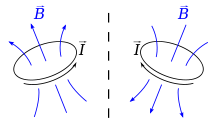Magnetic field, like all pseudovectors, changes sign when reflected in a mirror: When a loop of wire (black), carrying a current is reflected in a mirror (dotted line), the magnetic field it generates (blue) is not simply reflected in the mirror; rather, it is reflected and reversed.

The complete set of Maxwell's equations then are:$\nabla \cdot \mathbf{B} = 0,$$\nabla \cdot \mathbf{E} = \frac{\rho}{\epsilon_0},$$\nabla \times \mathbf{B} = \mu_0\mathbf{J} + \mu_0 \varepsilon_0 \frac{\partial \mathbf{E}} {\partial t},$$\nabla \times \mathbf{E} = - \frac{\partial \mathbf{B}} {\partial t},$

where J = complete microscopic current density and ρ is the charge density.

Technically, B is a pseudovector (also called an axial vector) due to being defined by a vector cross product. Because of the right-hand rule, a current-carrying loop viewed in a mirror results in a B vector that is both mirror-imaged and flipped in orientation, whereas an ordinary vector (e.g., velocity) is mirror-imaged only. (See diagram to right.)

As discussed above, materials respond to an applied electric E field and an applied magnetic B field by producing their own internal 'bound' charge and current distributions that contribute to E and B but are difficult to calculate. To circumvent this problem the auxiliary H and D fields are defined so that Maxwell's equations can be re-factored in terms of the free current density Jf and free charge density ρf:$\nabla \cdot \mathbf{B} = 0,$$\nabla \cdot \mathbf{D} = \rho_f,$$\nabla \times \mathbf{H} = \mathbf{J}_f + \frac{\partial \mathbf{D}} {\partial t},$$\nabla \times \mathbf{E} = - \frac{\partial \mathbf{B}} {\partial t}.$

These equations are not any more general than the original equations (if the 'bound' charges and currents in the material are known'). They also need to be supplemented by the relationship between B and H as well as that between E and D. On the other hand, for simple relationships between these quantities this form of Maxwell's equations can circumvent the need to calculate the bound charges and currents.

### Electric and magnetic fields: different aspects of the same phenomenon

According to the special theory of relativity, the partition of the electromagnetic force into separate electric and magnetic components is not fundamental, but varies with the observational frame of reference: An electric force perceived by one observer may be perceived by another (in a different frame of reference) as a magnetic force, or a mixture of electric and magnetic forces.

Formally, special relativity combines the electric and magnetic fields into a rank-2 tensor, called the electromagnetic tensor. Changing reference frames mixes these components. This is analogous to the way that special relativity mixes space and time into spacetime, and mass, momentum and energy into four-momentum.

### Magnetic vector potential

In advanced topics such as quantum mechanics and relativity it is often easier to work with a potential formulation of electrodynamics rather than in terms of the electric and magnetic fields. In this representation, the vector potential, A, and the scalar potential, φ, are defined such that:$\mathbf{B} = \nabla \times \mathbf{A},$$\mathbf{E} = - \nabla \varphi - \frac { \partial \mathbf{A} } { \partial t }.$

The vector potential A may be interpreted as a generalized potential momentum per unit charge just as φ is interpreted as a generalized potential energy per unit charge.

Maxwell's equations when expressed in terms of the potentials can be cast into a form that agrees with special relativity with little effort. In relativity A together with φ forms the four-potential analogous to the four-momentum which combines the momentum and energy of a particle. Using the four potential instead of the electromagnetic tensor has the advantage of being much simpler; further it can be easily modified to work with quantum mechanics.

### Quantum electrodynamics

In modern physics, the electromagnetic field is understood to be not a classical field, but rather a quantum field; it is represented not as a vector of three numbers at each point, but as a vector of three quantum operators at each point. The most accurate modern description of the electromagnetic interaction (and much else) is Quantum electrodynamics (QED), which is incorporated into a more complete theory known as the "Standard Model of particle physics".

In QED, the magnitude of the electromagnetic interactions between charged particles (and their antiparticles) is computed using perturbation theory; these rather complex formulas have a remarkable pictorial representation as Feynman diagrams in which virtual photons are exchanged.

Predictions of QED agree with experiments to an extremely high degree of accuracy: currently about 10−12 (and limited by experimental errors); for details see precision tests of QED. This makes QED one of the most accurate physical theories constructed thus far.

All equations in this article are in the classical approximation, which is less accurate than the quantum description mentioned here. However, under most everyday circumstances, the difference between the two theories is negligible.

## Important uses and examples of magnetic field

### Earth's magnetic field

The Earth's magnetic field is thought to be produced by convection currents in the outer liquid of Earth's core. The Dynamo theory proposes that these movements produce electric currents which, in turn, produce the magnetic field.

The presence of this field causes a compass, placed anywhere within it, to rotate so that the "north pole" of the magnet in the compass points roughly north, toward Earth's north magnetic pole. This is the traditional definition of the "north pole" of a magnet, although other equivalent definitions are also possible.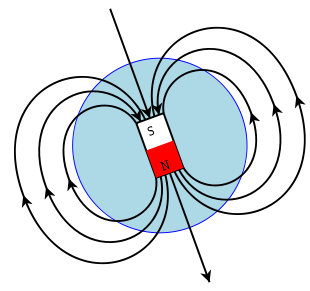A sketch of Earth's magnetic field representing the source of the field as a magnet. The geographic north pole of Earth is near the top of the diagram, the south pole near the bottom. The south pole of that magnet is deep in Earth's interior below Earth's North Magnetic Pole.

One confusion that arises from this definition is that, if Earth itself is considered as a magnet, the south pole of that magnet would be the one nearer the north magnetic pole, and vice-versa (opposite poles attract, so the north pole of the compass magnet is attracted to the south pole of Earth's interior magnet).

The north magnetic pole is so-named not because of the polarity of the field there but because of its geographical location. The north and south poles of a permanent magnet are so-called because they are "north-seeking" and "south-seeking", respectively.

The figure to the right is a sketch of Earth's magnetic field represented by field lines. For most locations, the magnetic field has a significant up/down component in addition to the North/South component. (There is also an East/West component; Earth's magnetic poles do not coincide exactly with Earth's geological pole.) The magnetic field can be visualised as a bar magnet buried deep in Earth's interior.

Earth's magnetic field is not constant — the strength of the field and the location of its poles vary. Moreover, the poles periodically reverse their orientation in a process called geomagnetic reversal. The most recent reversal occurred 780,000 years ago.

### Rotating magnetic fields

The rotating magnetic field is a key principle in the operation of alternating-current motors. A permanent magnet in such a field rotates so as to maintain its alignment with the external field. This effect was conceptualized by Nikola Tesla, and later utilized in his, and others', early AC (alternating-current) electric motors.

A rotating magnetic field can be constructed using two orthogonal coils with 90 degrees phase difference in their AC currents. However, in practice such a system would be supplied through a three-wire arrangement with unequal currents.

This inequality would cause serious problems in standardization of the conductor size and so, in order to overcome it, three-phase systems are used where the three currents are equal in magnitude and have 120 degrees phase difference. Three similar coils having mutual geometrical angles of 120 degrees create the rotating magnetic field in this case. The ability of the three-phase system to create a rotating field, utilized in electric motors, is one of the main reasons why three-phase systems dominate the world's electrical power supply systems.

Because magnets degrade with time, synchronous motors use DC voltage fed rotor windings which allows the excitation of the machine to be controlled and induction motors use short-circuited rotors (instead of a magnet) following the rotating magnetic field of a multicoiled stator. The short-circuited turns of the rotor develop eddy currents in the rotating field of the stator, and these currents in turn move the rotor by the Lorentz force.

In 1882, Nikola Tesla identified the concept of the rotating magnetic field. In 1885, Galileo Ferraris independently researched the concept. In 1888, Tesla gained U.S. Patent 381,968 for his work. Also in 1888, Ferraris published his research in a paper to the Royal Academy of Sciences in Turin.

### Hall effect

The charge carriers of a current carrying conductor placed in a transverse magnetic field experience a sideways Lorentz force; this results in a charge separation in a direction perpendicular to the current and to the magnetic field. The resultant voltage in that direction is proportional to the applied magnetic field. This is known as the 'Hall effect'.

The Hall effect is often used to measure the magnitude of a magnetic field. It is used as well to find the sign of the dominant charge carriers in materials such as semiconductors (negative electrons or positive holes).

### Magnetic circuits

An important use of H is in magnetic circuits where inside a linear material B = μ H. Here, μ is the magnetic permeability of the material. This result is similar in form to Ohm's law J = σ E, where J is the current density, σ is the conductance and E is the electric field. Extending this analogy, the counterpart to the macroscopic Ohm's law ( I = V ⁄ R ) is:$\Phi = \frac F R_m,$

where$\Phi = \int \mathbf{B}\cdot d\mathbf{A}$ is the magnetic flux in the circuit,$F = \int \mathbf{H}\cdot d\mathbf{l}$ is the magnetomotive force applied to the circuit, and Rm is the reluctance of the circuit. Here the reluctance Rm is a quantity similar in nature to resistance for the flux.

Using this analogy it is straight-forward to calculate the magnetic flux of complicated magnetic field geometries, by using all the available techniques of circuit theory.

### Magnetic field shape descriptions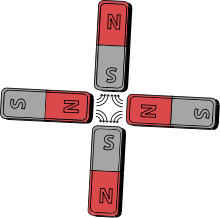Schematic quadrupole magnet ("four-pole") magnetic field. There are four steel pole tips, two opposing magnetic north poles and two opposing magnetic south poles.
• An azimuthal magnetic field is one that runs east-west.
• A meridional magnetic field is one that runs north-south. In the solar dynamo model of the Sun, differential rotation of the solar plasma causes the meridional magnetic field to stretch into an azimuthal magnetic field, a process called the omega-effect. The reverse process is called the alpha-effect.
• A dipole magnetic field is one seen around a bar magnet or around a charged elementary particle with nonzero spin.
• A quadrupole magnetic field is one seen, for example, between the poles of four bar magnets. The field strength grows linearly with the radial distance from its longitudinal axis.
• A solenoidal magnetic field is similar to a dipole magnetic field, except that a solid bar magnet is replaced by a hollow electromagnetic coil magnet.
• A toroidal magnetic field occurs in a doughnut-shaped coil, the electric current spiraling around the tube-like surface, and is found, for example, in a tokamak.
• A poloidal magnetic field is generated by a current flowing in a ring, and is found, for example, in a tokamak.
• A radial magnetic field is one in which the field lines are directed from the center outwards, similar to the spokes in a bicycle wheel. An example can be found in a loudspeaker transducers (driver).
• A helical magnetic field is corkscrew-shaped, and sometimes seen in space plasmas such as the Orion Molecular Cloud.

### Magnetic dipoles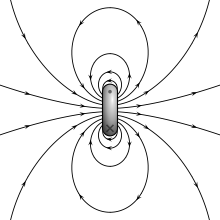Magnetic field lines around a ”magnetostatic dipole” pointing to the right.

The magnetic field of a magnetic dipole is depicted on the right. From outside, the ideal magnetic dipole is identical to that of an ideal electric dipole of the same strength. Unlike the electric dipole, a magnetic dipole is properly modeled as a current loop having a current I and an area a. Such a current loop has a magnetic moment of:$m=Ia, \,$

where the direction of m is perpendicular to the area of the loop and depends on the direction of the current using the right-hand rule. An ideal magnetic dipole is modeled as a real magnetic dipole whose area a has been reduced to zero and its current I increased to infinity such that the product m = Ia is finite. In this model it is easy to see the connection between angular momentum and magnetic moment which is the basis of the Einstein-de Haas effect "rotation by magnetization" and its inverse, the Barnett effect or "magnetization by rotation". Rotating the loop faster (in the same direction) increases the current and therefore the magnetic moment, for example.

It is sometimes useful to model the magnetic dipole similar to the electric dipole with two equal but opposite magnetic charges (one south the other north) separated by distance d. This model produces an H-field not a B-field. Such a model is deficient, though, both in that there are no magnetic charges and in that it obscures the link between electricity and magnetism. Further, as discussed above it fails to explain the inherent connection between angular momentum and magnetism.

General

Mathematics

Applications

• Dynamo theory – a proposed mechanism for the creation of the Earth's magnetic field.
• Helmholtz coil – a device for producing a region of nearly uniform magnetic field.
• Magnetic field viewing film – Film used to view the magnetic field of an area.
• Maxwell coil – a device for producing a large volume of an almost constant magnetic field.
• Stellar magnetic field – a discussion of the magnetic field of stars.
• Teltron Tube – device used to display an electron beam and demonstrates effect of electric and magnetic fields on moving charges.

Wikimedia Foundation. 2010.

### Look at other dictionaries:

• Magnetic field — Field Field (f[=e]ld), n. [OE. feld, fild, AS. feld; akin to D. veld, G. feld, Sw. f[ a]lt, Dan. felt, Icel. fold field of grass, AS. folde earth, land, ground, OS. folda.] 1. Cleared land; land suitable for tillage or pasture; cultivated ground; …   The Collaborative International Dictionary of English

• magnetic field — n. (Physics) The space around a magnet through which it exerts magnetic force; a field of force surrounding a permanent magnet, electrical current, or a moving charged particle; called also {magnetic flux} and {field of magnetic force}. [WordNet… …   The Collaborative International Dictionary of English

• magnetic field — n the portion of space near a magnetic body or a current carrying body in which the magnetic forces due to the body or current can be detected <the magnetic field of the heart> * * * that portion of space about a magnet in which its action… …   Medical dictionary

• magnetic field — magnetic fields N COUNT A magnetic field is an area around a magnet, or something functioning as a magnet, in which the magnet s power to attract things is felt …   English dictionary

• magnetic field — n. a physical field that arises from an electric charge in motion, producing a force on a moving electric charge …   English World dictionary

• magnetic Field —   [engl.], Magnetfeld …   Universal-Lexikon

• magnetic field — ► NOUN ▪ a region around a magnet within which the force of magnetism acts …   English terms dictionary

• magnetic field — 1. a region of space near a magnet, electric current, or moving charged particle in which a magnetic force acts on any other magnet, electric current, or moving charged particle. 2. See magnetic intensity. [1835 45] * * * Region around a magnet,… …   Universalium

• magnetic field — noun the lines of force surrounding a permanent magnet or a moving charged particle (Freq. 3) • Syn: ↑magnetic flux, ↑flux • Hypernyms: ↑field, ↑field of force, ↑force field • Hyponyms …   Useful english dictionary

• magnetic field — UK / US noun [countable] Word forms magnetic field : singular magnetic field plural magnetic fields physics an area that the power of a magnet affects …   English dictionary